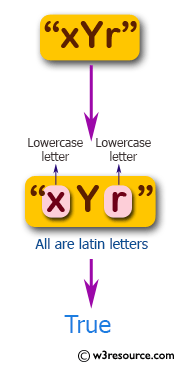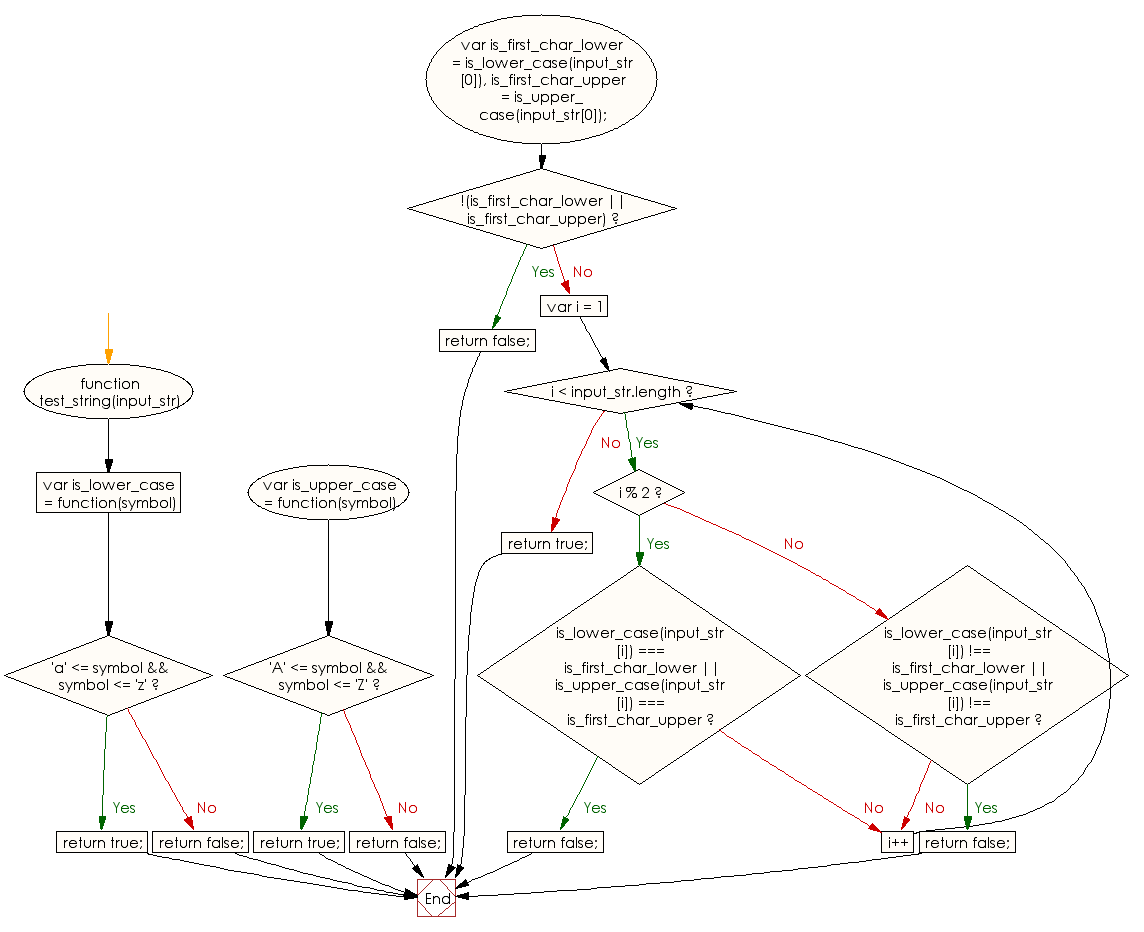# JavaScript: Check whether a given string contains only Latin letters and no two uppercase and no two lowercase letters are in adjacent positions

## JavaScript Basic: Exercise-101 with Solution

Write a JavaScript program to check whether a given string contains only Latin letters and no two uppercase and no two lowercase letters are in adjacent positions.

Pictorial Presentation:Sample Solution:

HTML Code:

``````<!DOCTYPE html>
<html>
<meta charset="utf-8">
<meta name="viewport" content="width=device-width">
<title> Check whether a given string contains only Latin letters and no two uppercase and no two lowercase letters are in adjacent positions</title>
<body>

</body>
</html>
```
```

JavaScript Code:

``````function test_string(input_str) {

var is_lower_case = function(symbol) {
if ('a' <= symbol && symbol <= 'z') {
return true;
}
return false;
}

var is_upper_case = function(symbol) {
if ('A' <= symbol && symbol <= 'Z') {
return true;
}
return false;
}

var is_first_char_lower = is_lower_case(input_str),
is_first_char_upper = is_upper_case(input_str);

if (!(is_first_char_lower || is_first_char_upper)) {
return false;
}

for (var i = 1; i < input_str.length; i++) {
if (i % 2) {
if (is_lower_case(input_str[i]) === is_first_char_lower ||
is_upper_case(input_str[i]) === is_first_char_upper) {
return false;
}
} else {
if (is_lower_case(input_str[i]) !== is_first_char_lower ||
is_upper_case(input_str[i]) !== is_first_char_upper) {
return false;
}
}
}

return true;
}

console.log(test_string('xYr'));
console.log(test_string('XXyx'));
```
```

Sample Output:

```true
false
```

Flowchart:ES6 Version:

``````function test_string(input_str) {
const is_lower_case = symbol => {
if ('a' <= symbol && symbol <= 'z') {
return true;
}
return false;
};

const is_upper_case = symbol => {
if ('A' <= symbol && symbol <= 'Z') {
return true;
}
return false;
};

const is_first_char_lower = is_lower_case(input_str);
const is_first_char_upper = is_upper_case(input_str);

if (!(is_first_char_lower || is_first_char_upper)) {
return false;
}

for (let i = 1; i < input_str.length; i++) {
if (i % 2) {
if (is_lower_case(input_str[i]) === is_first_char_lower ||
is_upper_case(input_str[i]) === is_first_char_upper) {
return false;
}
} else {
if (is_lower_case(input_str[i]) !== is_first_char_lower ||
is_upper_case(input_str[i]) !== is_first_char_upper) {
return false;
}
}
}

return true;
}

console.log(test_string('xYr'));
console.log(test_string('XXyx'));
``````

Live Demo:

See the Pen javascript-basic-exercise-101 by w3resource (@w3resource) on CodePen.

What is the difficulty level of this exercise?

﻿

## JavaScript: Tips of the Day

Promise object

```const myPromise = () => Promise.resolve('I have resolved!');

function firstFunction() {
myPromise().then(res => console.log(res));
console.log('second');
}

async function secondFunction() {
console.log(await myPromise());
console.log('second');
}

firstFunction();
secondFunction();
```

With a promise, we basically say I want to execute this function, but I'll put it aside for now while it's running since this might take a while. Only when a certain value is resolved (or rejected), and when the call stack is empty, I want to use this value.
We can get this value with both .then and the await keyword in an async function. Although we can get a promise's value with both .then and await, they work a bit differently.
In the firstFunction, we (sort of) put the myPromise function aside while it was running, but continued running the other code, which is console.log('second') in this case. Then, the function resolved with the string I have resolved, which then got logged after it saw that the callstack was empty.
With the await keyword in secondFunction, we literally pause the execution of an async function until the value has been resolved before moving to the next line.
This means that it waited for the myPromise to resolve with the value I have resolved, and only once that happened, we moved to the next line: second got logged.

Ref: https://bit.ly/3jFRBje